A two-dimensional continuum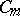,, in the four-dimensional Euclidean spacesuch that its homological dimension modulo the given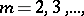is. In this sense these continua are "dimensionally deficient" . L.S. Pontryagin  has constructed surfaces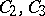such that their topological product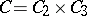is a continuum of dimension. Thus, the conjecture stating that under topological multiplication of two (metric) compacta their dimensions are added, was disproved. He proved this conjecture for homological dimensions modulo a prime number and, in general, over any group of coefficients which is a field. In  a two-dimensional continuumin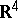has been constructed whose topological square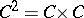is three-dimensional.